# Understanding Kinect V2 Joints and Coordinate System

One of the most frequent questions I get from people working with the Kinect, and Kinectron is: what is the skeleton actually made up of, and how is that represented? Although many people have written about this, I haven’t been able to find one place that has all the info I usually end up sharing in one place, so I’ve decided to compile it all here.

### The Kinect Skeleton System

Before I get started, it’s important to note that everything in this post is about the Kinect for Xbox One, what most people call the Kinect Version 2.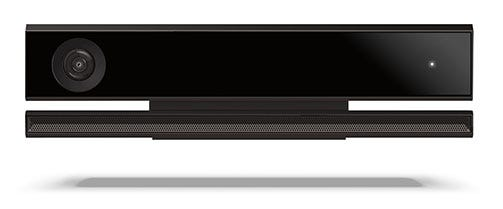Kinect for Xbox One (aka Kinect V2). Image from MS Developer blog.

The Kinect can track up to six skeletons at one time. Each of these skeletons has 25 joints.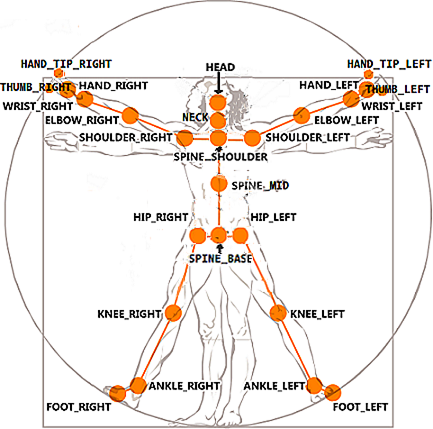Kinect v2 25-joint skeleton. Image from Stack Overflow.

Note that the Kinect skeleton returns “joints” not “bones.” It’s an important distinction that has big implications when you think about how bodies move in space. The joints are numbered 0–24. (Protip: if you’re working with Kinectron, you can access these by their names.)

Each joint has 11 properties: color (x, y); depth (x, y); camera (x, y, z); and orientation( x, y, z, w).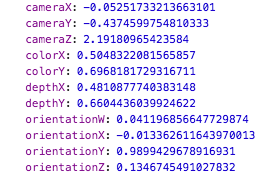An example of the 11 properties of one Kinect joint in the Javascript console.

Color Coordinates (X, Y)

The Kinect has two cameras—a color (RGB) camera and a depth camera—and they have different resolutions. The color camera is 1920 x 1080. The depth camera is 512 x 424. The color coordinates (x, y) and depth coordinates (x, y) take this into account.

The color coordinates (x, y) are the coordinates of the joint on the image from the color camera. Here’s the explanation from the SDK:

A color space point describes a 2D point on the color image. So a position in color space is a row/column location of a pixel on the image, where x=0, y=0 is the pixel at the top left of the color image, and x=1919, y=1079 (width-1, height-1) corresponds to the bottom right.

Simply put, the Kinect returns a value between 0 and 1 for the color coordinates (x, y). The values are percentages of a set scale of 0–1 based on the camera’s resolution, 1920 x 1080. If you’re drawing a skeleton to a 2D canvas (like a p5.js canvas), an easy way to make sure that the joints are positioned correctly is to make the canvas the same resolution as the camera, and map the coordinates (x, y) based on the width and height of the canvas.

Let’s use the values from the joint example above to understand what they represent. In this example colorX is 0.5048322081565857 and colorY is 0.6968181729316711. As we know, the color image is 1920 x 1080. In order to draw the joint in the proper place on the color image, multiply the color values (x, y) by the width and height of the image. So, the x value is 0.5048322081565857 x 1920 and the y value is 0.6968181729316711 x 1080. So, x is approximately 969 and y is approximately 753.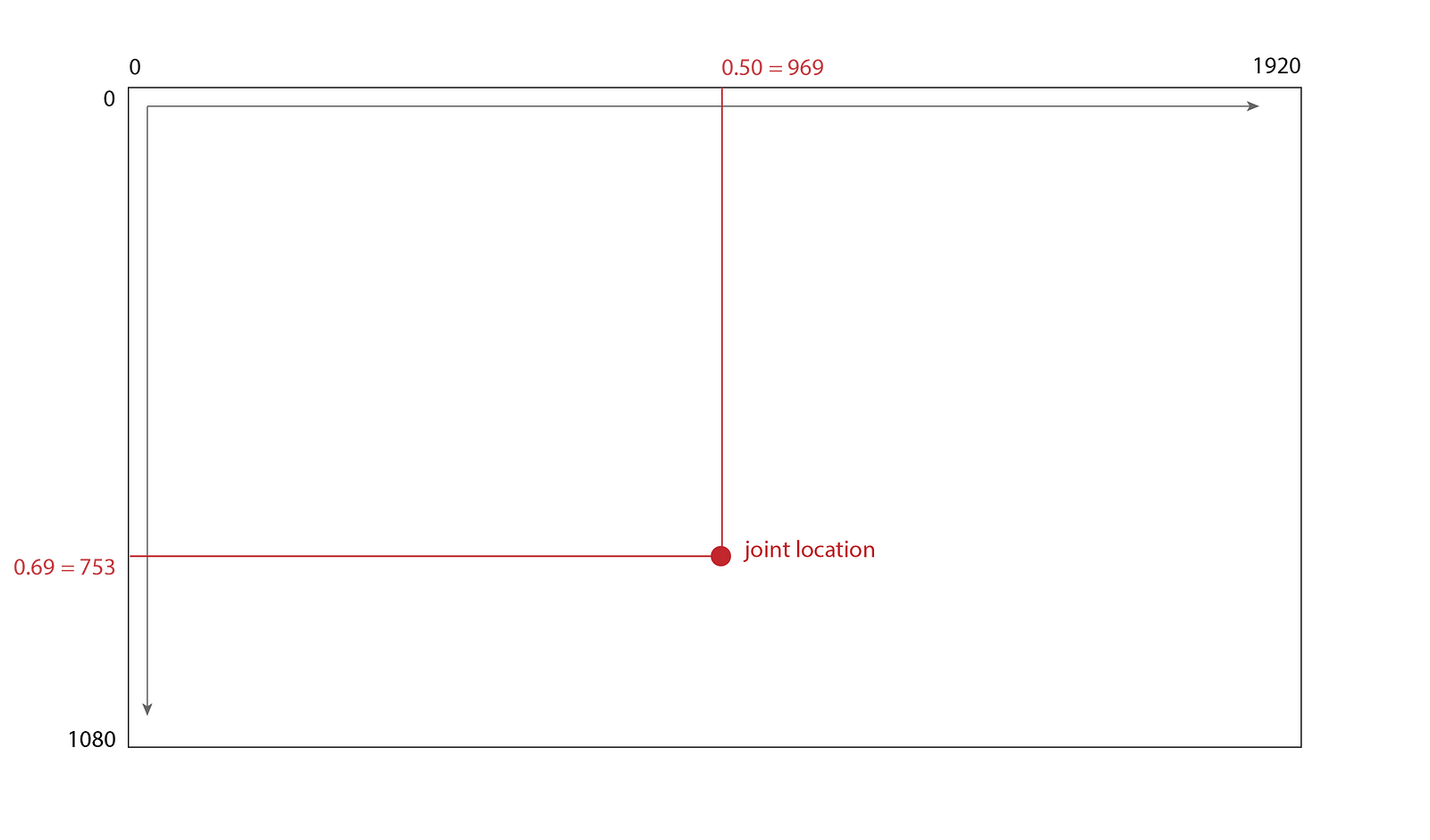Kinect color coordinates (x, y) mapping example.

Depth Coordinates (X, Y)

The depth coordinates (x, y) are the coordinates of the joint on the image from the depth camera. Here’s the explanation from the SDK:

Depth space is the term used to describe a 2D location on the depth image. Think of this as a row/column location of a pixel where x is the column and y is the row. So x=0, y=0 corresponds to the top left corner of the image and x=511, y=423 (width-1, height-1) is the bottom right corner of the image.

Just like colorX and colorY, the Kinect returns a value between 0 and 1 for the depth coordinates (x, y).

Let’s again use the values from the joint example above to understand what the values represent. In this example depthX is 0.4810877740383148 and depthY is 0.6604436039924622. The depth image resolution is 512 x 424. In order to draw the joint in the proper place on the depth image, multiply the depth values (x, y) by the width and height of the image. So, the x value is 0.4810877740383148 x 512 and the y value is 0.6604436039924622 x 424. So, x is approximately 246 and y is 280.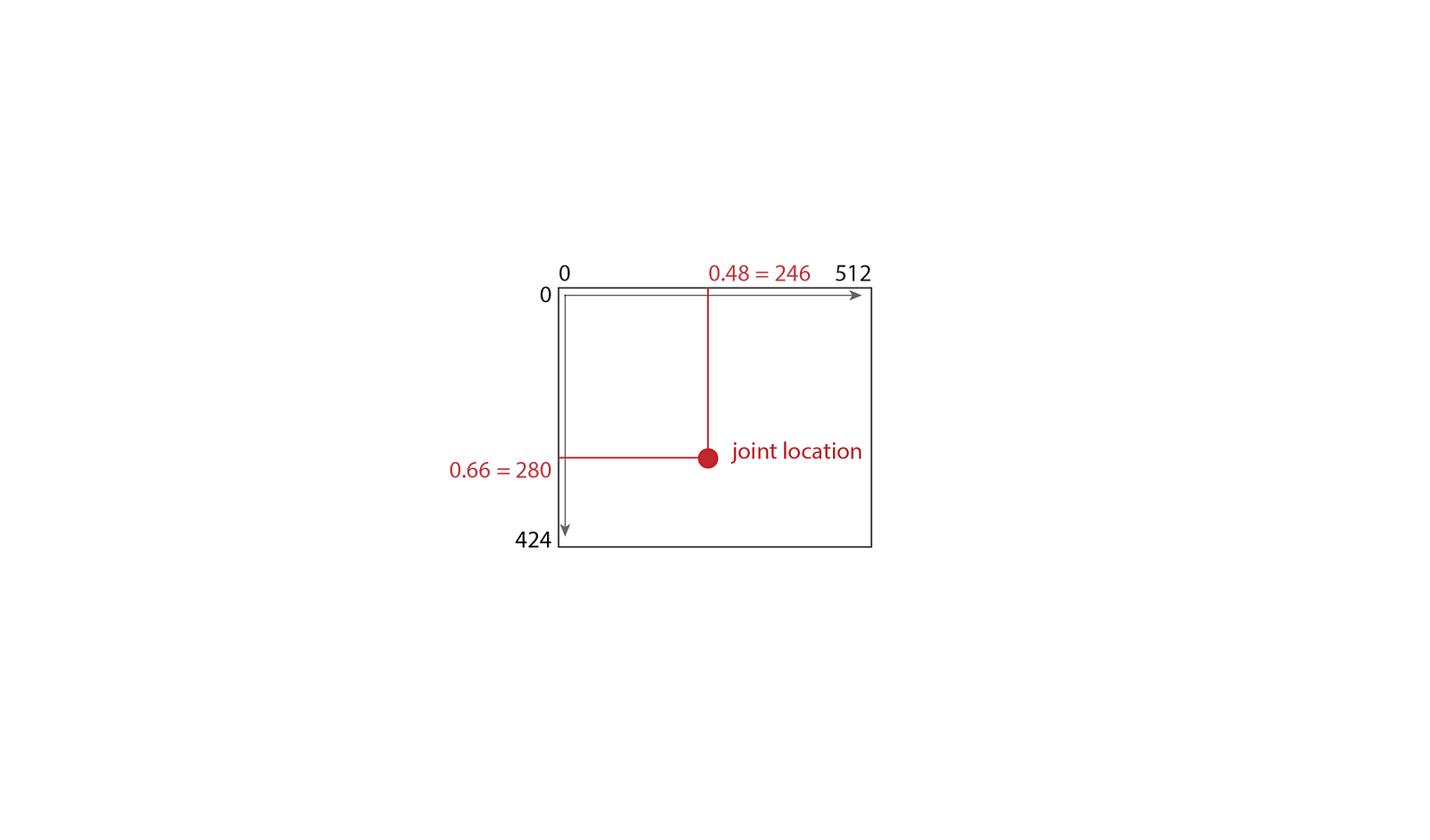Kinect depth coordinates (x, y) mapping example. The depth image is drawn to scale with color image above.

Camera Coordinates (X, Y, Z, W)

The Kinect’s camera coordinates use the Kinect’s infrared sensor to find 3D points of the joints in space. These are the coordinates to use for joint positioning in 3D projects. The camera space coordinates are handled differently from the color and depth coordinates. From the SDK:

Camera space refers to the 3D coordinate system used by Kinect. The coordinate system is defined as follows:
The origin (x=0, y=0, z=0) is located at the center of the IR sensor on Kinect
X grows to the sensor’s left [from the sensor’s POV]
Y grows up (note that this direction is based on the sensor’s tilt)
Z grows out in the direction the sensor is facing
1 unit = 1 meter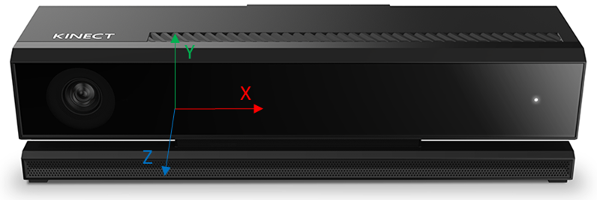Camera space coordinates from the Kinect SDK.

In camera space, the coordinates are measured in meters. The coordinates (x, y) can be positive or negative, as they extend in both direction from the sensor. The z coordinate will always be positive, as it grows out from the sensor.

The depth range of the Kinect is eight meters, but the skeleton tracking range is 0.5m to 4.5m, and it has trouble finding a skeleton at closer than 1.5m because of the field of view of the camera. So the cameraZ value will usually fall somewhere between 1.5 and 4.5.

The x coordinate can be negative or positive, because 0 is at the center of the sensor, and joints can be tracked to the sensor’s left (positive) or right (negative). The range of cameraX depends on the joint’s distance from the camera, but it can go up to about six meters in width. (See Kinect V2 Maximum Range discussion for more on this.)

The y coordinate can also be negative or positive: the value is positive when it is above the sensor, and negative when it is below the sensor. The cameraY range will depend on the distance from the camera, but can sense about five meters in height.

We’ll use the values from the joint example above to understand what the camera values represent. In this example cameraX is -0.05251733213663101, cameraY is -0.4374599754810333 and cameraZ is 2.19180965423584. The x and y values are negative, which means the joint is about 0.05 meters to the right of the sensor, 0.43 meters below the sensor and 2.19 meters in front of the sensor.

It’s important to note the difference between the measurements used in the 2D (color and depth) coordinates and the 3D (camera) coordinates. The color and depth values are delivered as percentages of a set scale of 0–1 at their respective resolutions. In camera space, the three values—camera coordinates (x, y, z)—use the same units—meters—and can be scaled evenly to keep their proportions. For example, multiplying each of the values by 1000 will return a point at approximately -53, -437, 2191 (rounded to integers for ease of typing ;) ). The distances are now in millimeters, which are more convenient for translating to pixel space.

Orientation Coordinates (X, Y, Z, W)

Kinect uses quaternions to deliver joint orientation. A common pitfall here is to assume that orientation coordinates (x, y, z) are equal to yaw, pitch and roll, and coordinate w can be conveniently discarded. In fact, quaternions are a 4D way to store the 3D orientation, and they need to be converted to be useful.

Here is the best explanation I’ve been able to find of quaternions, written by Pete D:

[Quaternions] are a way to describe an orientation in 3d space and are used to avoid gimbal-lock related problems, which arise from using Euler angles for rotation. They provide a great way to store and animate rotations, but ultimately are converted back to matrix form and your graphics programming environment most-likely provides functions to do this.

This is unfortunately where my practical knowledge of 4D math ends :P but I will write a post soon about my experience trying to use the Kinect orientation quaternions for avateering.

I wrote this article as a response to questions from creative coders using the open source software, Kinectron. Kinectron is a realtime peer server for Kinect V2 that makes skeletal and volumetric data available in the browser through an easy to use API.

Kinectron was developed under the Google Experiments in Storytelling (xStory) Grant at New York University’s Interactive Telecommunications Program (ITP). It is currently under development. Please get in touch if you’d like to contribute.

Many thanks to Aarón Montoya-Moraga for all the helpful insights, and for his unwavering commitment to open source creative tools.